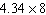Name:    Quiz 3.1-3.3

Multiple Choice
Identify the choice that best completes the statement or answers the question.

Estimate by rounding each factor to the nearest whole number.

1.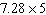a. 35 c. 30 b. 40 d. 36.5

Estimate the area of the figure by rounding to the nearest whole number.

2.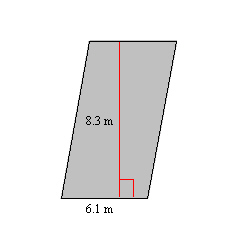a. 54 m2 c. 42 m2 b. 49 m2 d. 48 m2

3.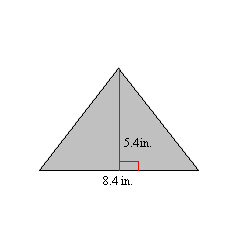a. 20.5 in.2 c. 28 in.2 b. 24 in.2 d. 20 in.2

Use the Distributive Property to rewrite the expression.

4.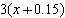a. 3x + 0.45 c. 3x + 0.15 b. 3.15x d. 0.45x

5.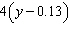a. 0.52y c. 4y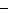0.52 b. 4.13y d. 4y0.13

Evaluate the expression.

6.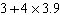a. 18.6 c. 15.9 b. 15.7 d. 27.3

7.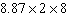a. 25.74 c. 86.96 b. 141.92 d. 72.96

Evaluate the expression when x = 1.4 and y = 4.21.

8.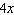a. 4 c. 16.84 b. 5.6 d. 0.56

9.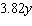a. 16.0822 c. 5.348 b. 17.0822 d. 1.6082

10.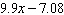a. 34.699 c. 6.79 b. 34.599 d. 6.78

Convert the fractions or mixed numbers to decimals. Then multiply.

11.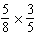a. 0.375 c. 0.0375 b. 0.1375 d. 0.475

12.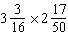a. 7.55875 c. 7.45875 b. 0.745875 d. 0.845875

Numeric Response

Find the product. Use an estimate to place the decimal point.

1.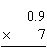2.